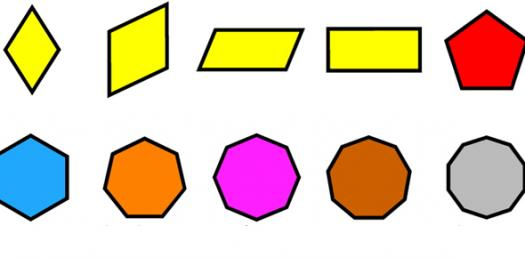# 6.1 And 6.2 Quiz - Polygons

12 Questions | Attempts: 336
ShareSettingsVocabulary and how to find the interior and exterior angle sum of polygons

• 1.
How many sides does a do-degagon have?
• A.

10

• B.

12

• C.

8

• D.

6

• E.

5

• 2.
A regular polygon has which of the following?I. congruent sidesII. congruent anglesIII. same shape
• A.

I only

• B.

II only

• C.

III only

• D.

I and II only

• E.

I, II and III

• 3.
A stop sign has the same shape as which figure?
• A.

• B.

Pentagon

• C.

Hexagon

• D.

Heptagon

• E.

Octagon

• 4.
The figure below can be described by which of the following? I. regular polygon    II. convex polygon     III. pentagon
• A.

I only

• B.

II only

• C.

III only

• D.

I and II only

• E.

II and III only

• 5.
What is the interior angle sum of a pentagon?
• A.

180

• B.

360

• C.

540

• D.

720

• E.

900

• 6.
How many sides does a convex polygon have if it's interior angle sum is 900 degrees?
• A.

7

• B.

6

• C.

5

• D.

4

• E.

3

• 7.
What is the measure in degrees of each interior angle of a regular hexagon?
• A.

60

• B.

90

• C.

108

• D.

120

• E.

144

• 8.
What is the measure in degrees of each interior angle of a regular octagon?
• A.

144

• B.

135

• C.

120

• D.

108

• E.

90

• 9.
What is the exterior angle sum of a pentagon?
• A.

360

• B.

540

• C.

720

• D.

900

• E.

1080

• 10.
One exterior angle of a regular polygon is equal to 60 degrees. What is the shape of the figure?
• A.

Triangle

• B.

Square

• C.

Pentagon

• D.

Hexagon

• E.

Octagon

• 11.
One exterior angle of a regular polygon is 20 degrees. How many sides does it have?
• A.

9

• B.

18

• C.

12

• D.

36

• E.

4

• 12.
What is the measure in degrees of each exterior angle of a regular do-decagon?
• A.

30

• B.

60

• C.

90

• D.

120

• E.

180

## Related TopicsBack to top
×

Wait!
Here's an interesting quiz for you.